Courses
Courses for Kids
Free study material
Free LIVE classes
MoreA quadrilateral is a simple closed geometric figure with four sides and four angles. In simple words, a quadrilateral has four points lying in the same plane, out of which no three are collinear, and those points are joined by lines that do not intersect each other except at their common endpoints. A quadrilateral has 4 angles, 2 pairs of opposite sides and 4 pairs of adjacent sides each and a pair of diagonals.

Square, Rectangle, Kite, Rhombus, Parallelogram and Trapezium are examples of the quadrilateral.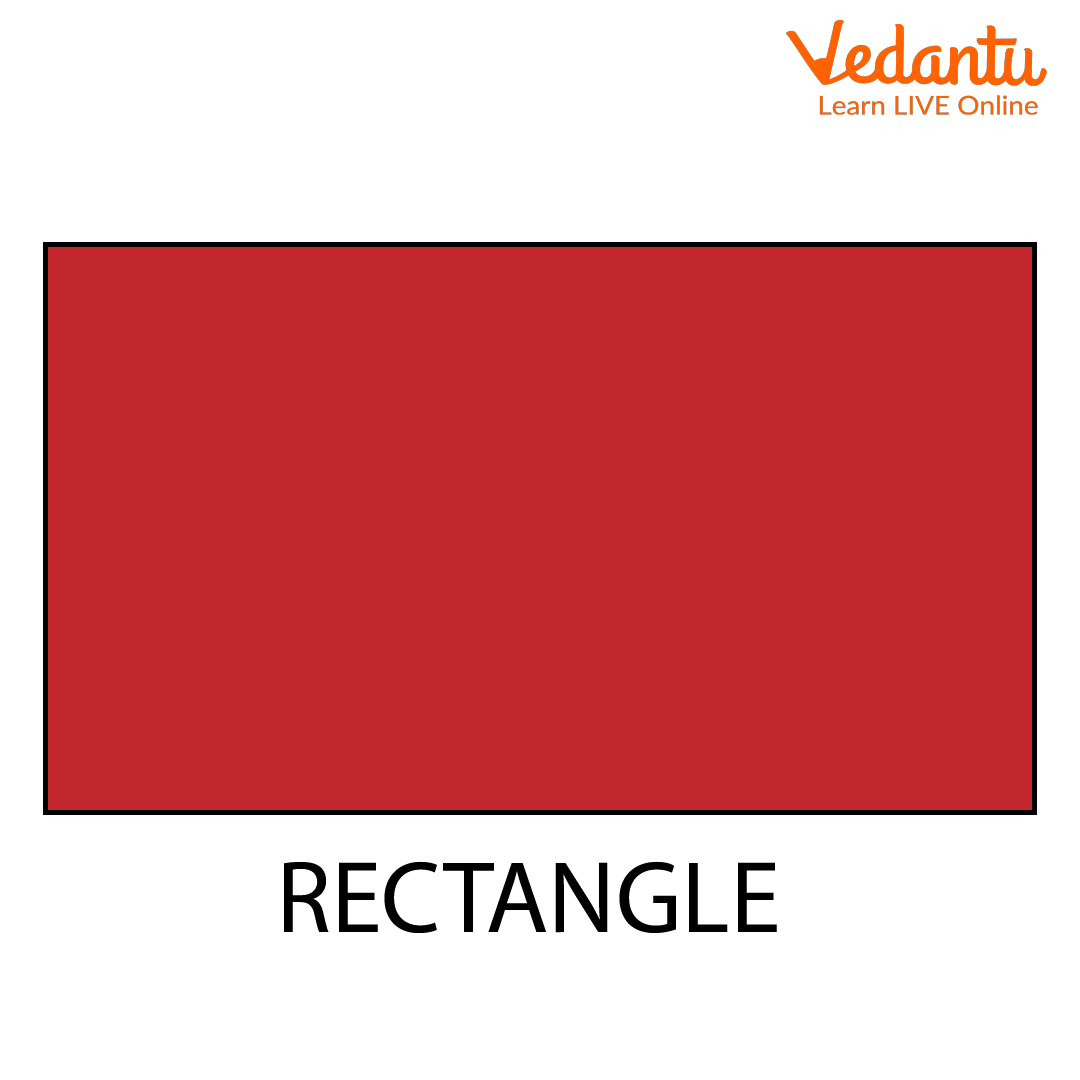A Rectangle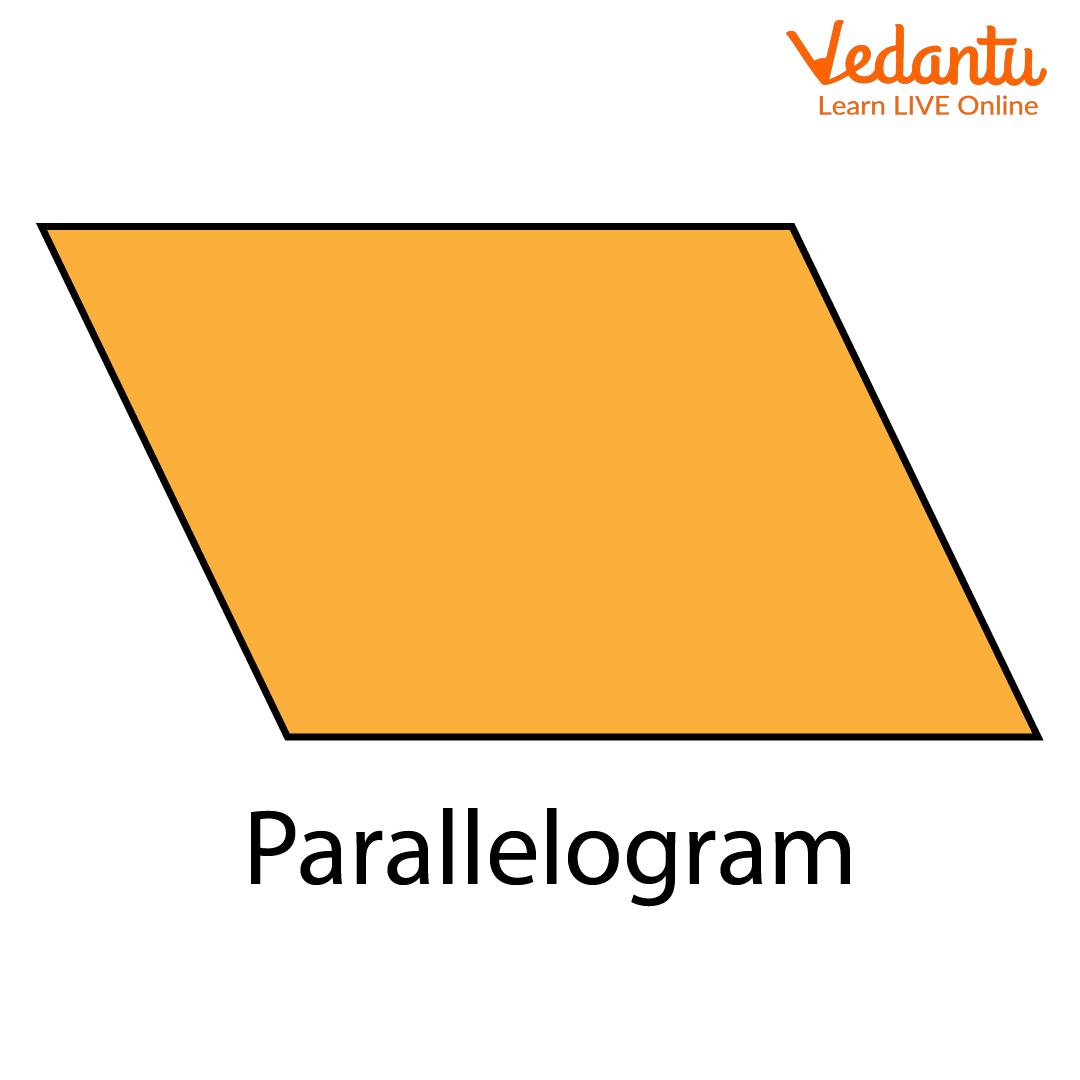A Parallelogram

### What are the Vertices of a Quadrilateral?

The common point joining the sides of a quadrilateral is said to be the vertices of a quadrilateral.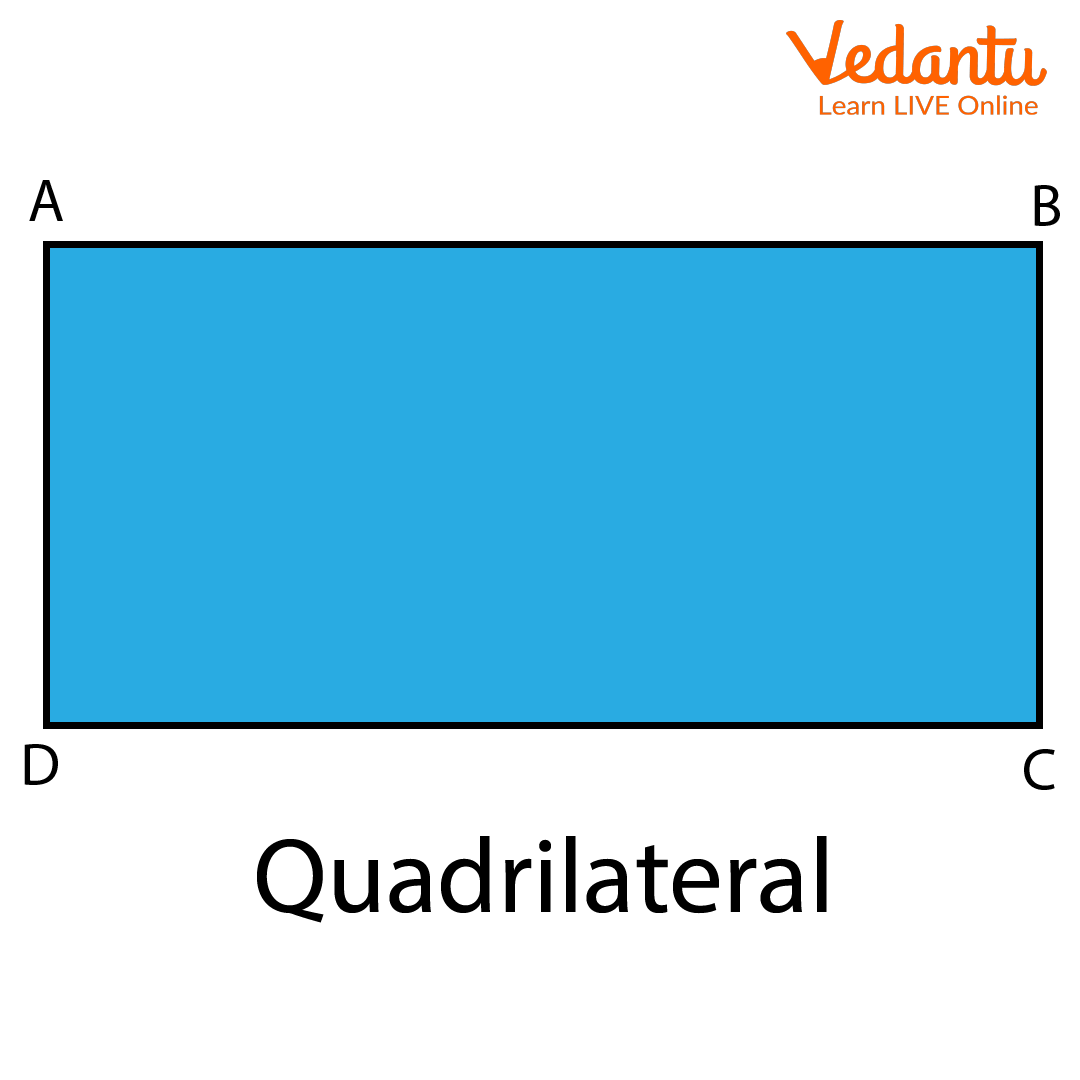In quadrilateral ABCD, shown in the above figure, A, B, C and D are the vertices.

What are the edges of a quadrilateral?

The line segments joining the vertices of the quadrilateral are said to be the edges or sides of that quadrilateral.

In the quadrilateral ABCD shown above, AB, BC, AD and CD are the edges.

### How many Diagonals does a Quadrilateral Have?

Let us see how many diagonals does quadrilateral have. The line segments joining the non-adjacent opposite vertices of a quadrilateral are called the diagonals of a quadrilateral. The diagonals of a quadrilateral intersect and bisect each other.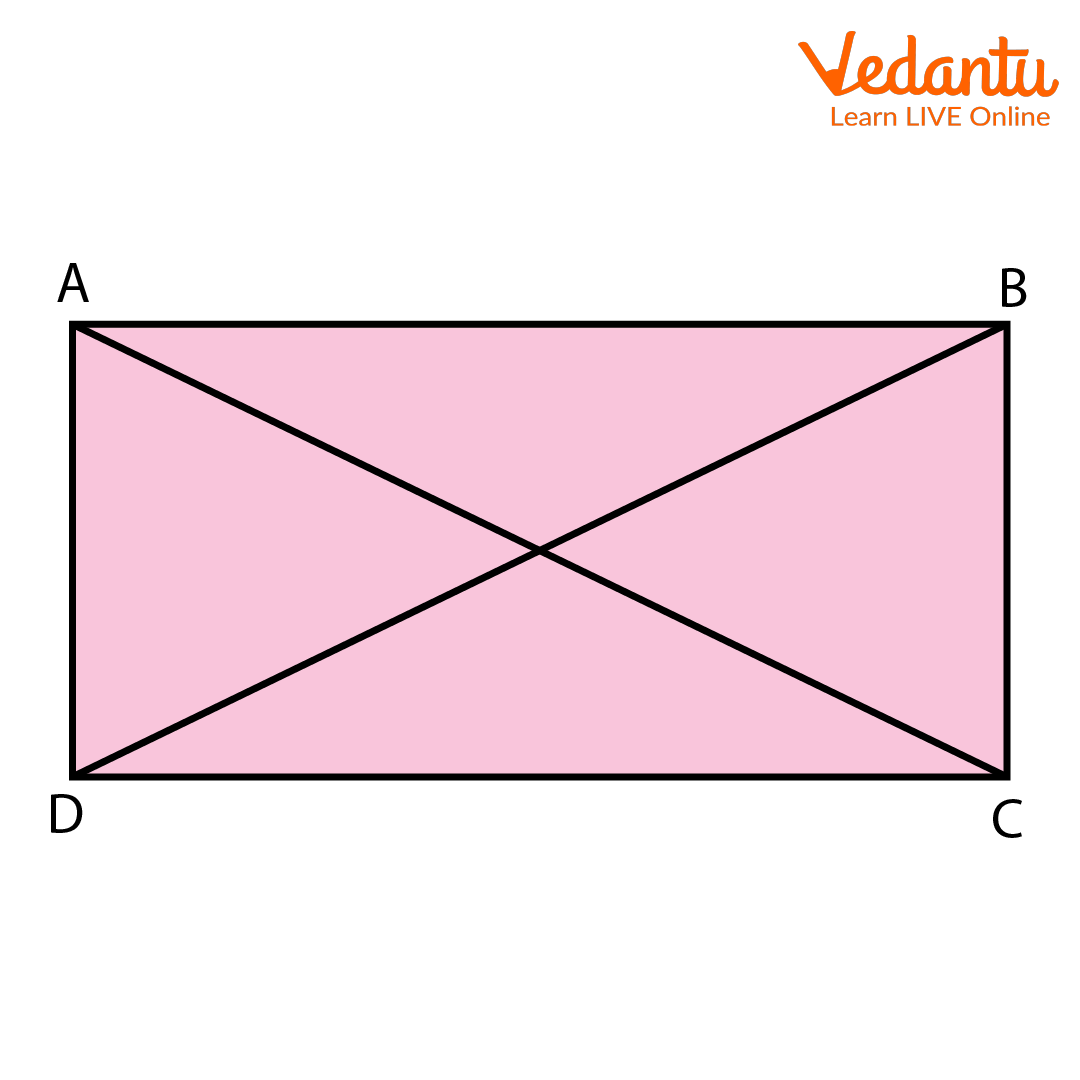In quadrilateral ABCD shown above, AC and BD are the diagonals.

### How many Angles are there in a Quadrilateral?

Let us see how many angles does a quadrilateral have? Angles of a quadrilateral refer to the angles formed at each vertex by the adjacent sides of the quadrilateral. A quadrilateral has 4 angles. The sum of a quadrilateral's angles is $360^\circ$.

In quadrilateral ABCD shown above, $\angle {\rm{A}}$,$\angle {\rm{B}}$,$\angle {\rm{C}}$ and $\angle {\rm{D}}$ are the angles of the quadrilateral.

The sides of a quadrilateral with a common endpoint are called its adjacent sides.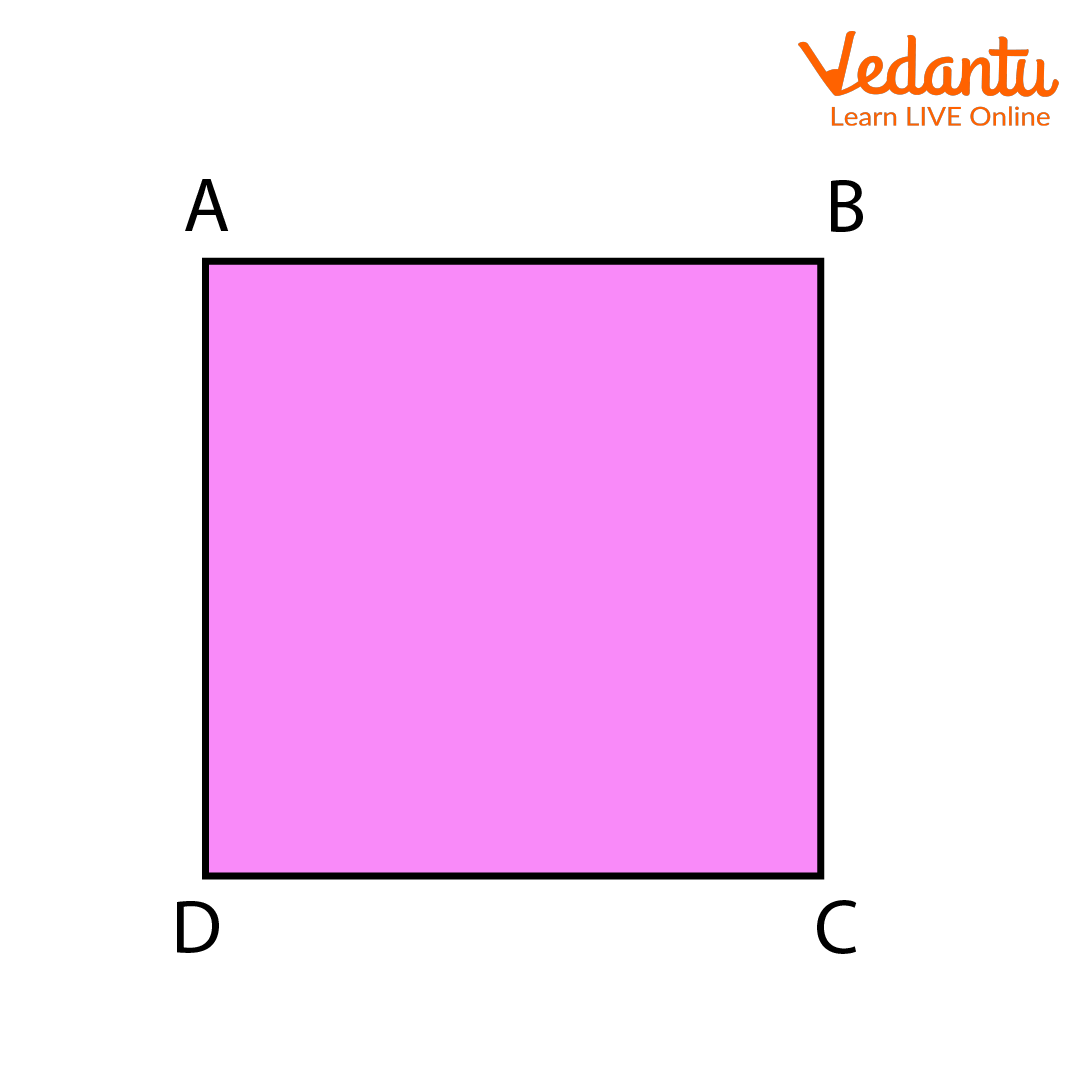In the given figure, (AB, BC), (BC, CD), (CD, DA) and (DA, AB) are four pairs of adjacent sides of quadrilateral ABCD.

### Solved Examples :

1. Identify the pairs of adjacent sides from the given figure.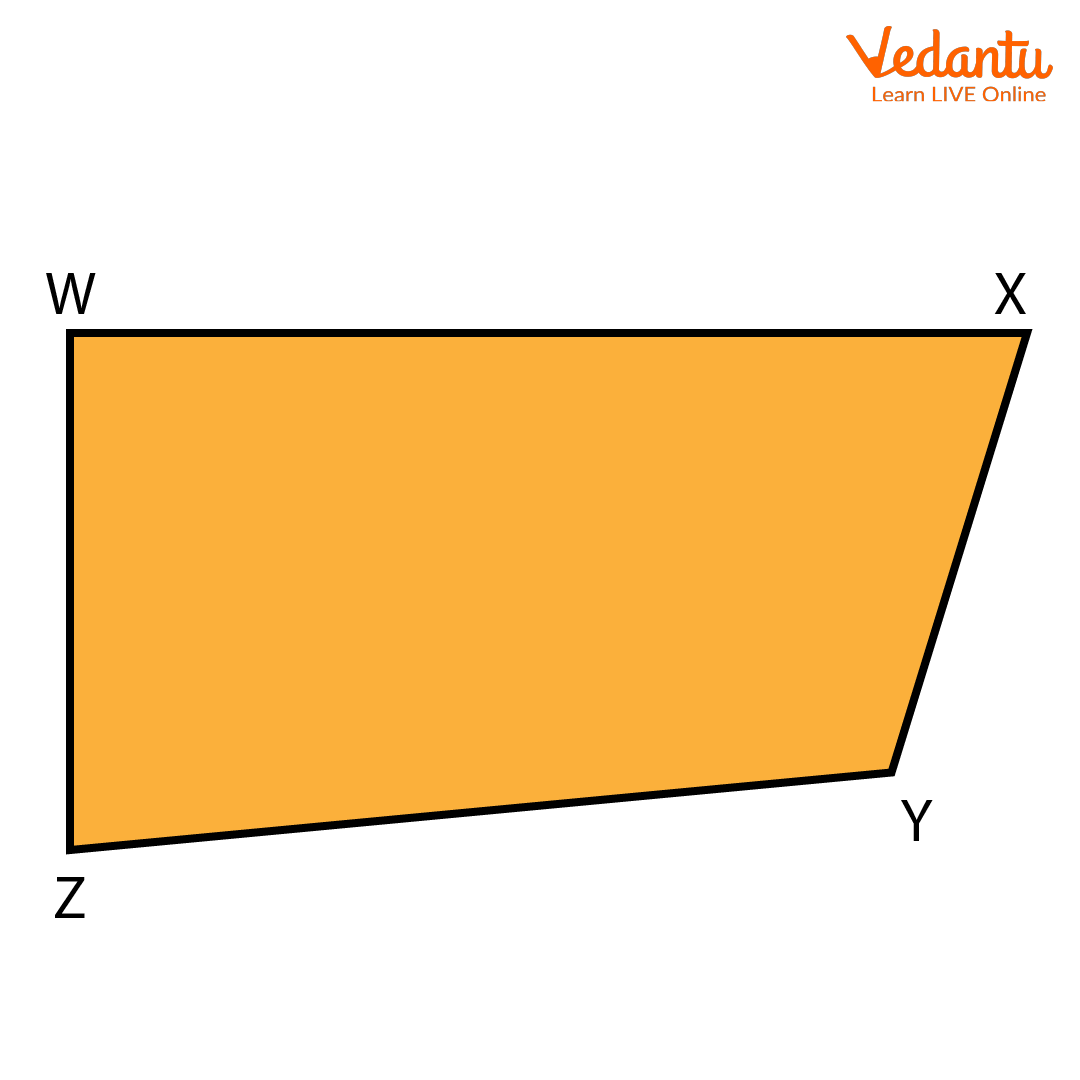Solution: In the given quadrilateral, WXYZ, (WX, XY), (XY, YZ), (YZ, ZW) and (ZW, WX) are the pairs of adjacent sides.

1. Identify the pairs of adjacent sides from the given figure.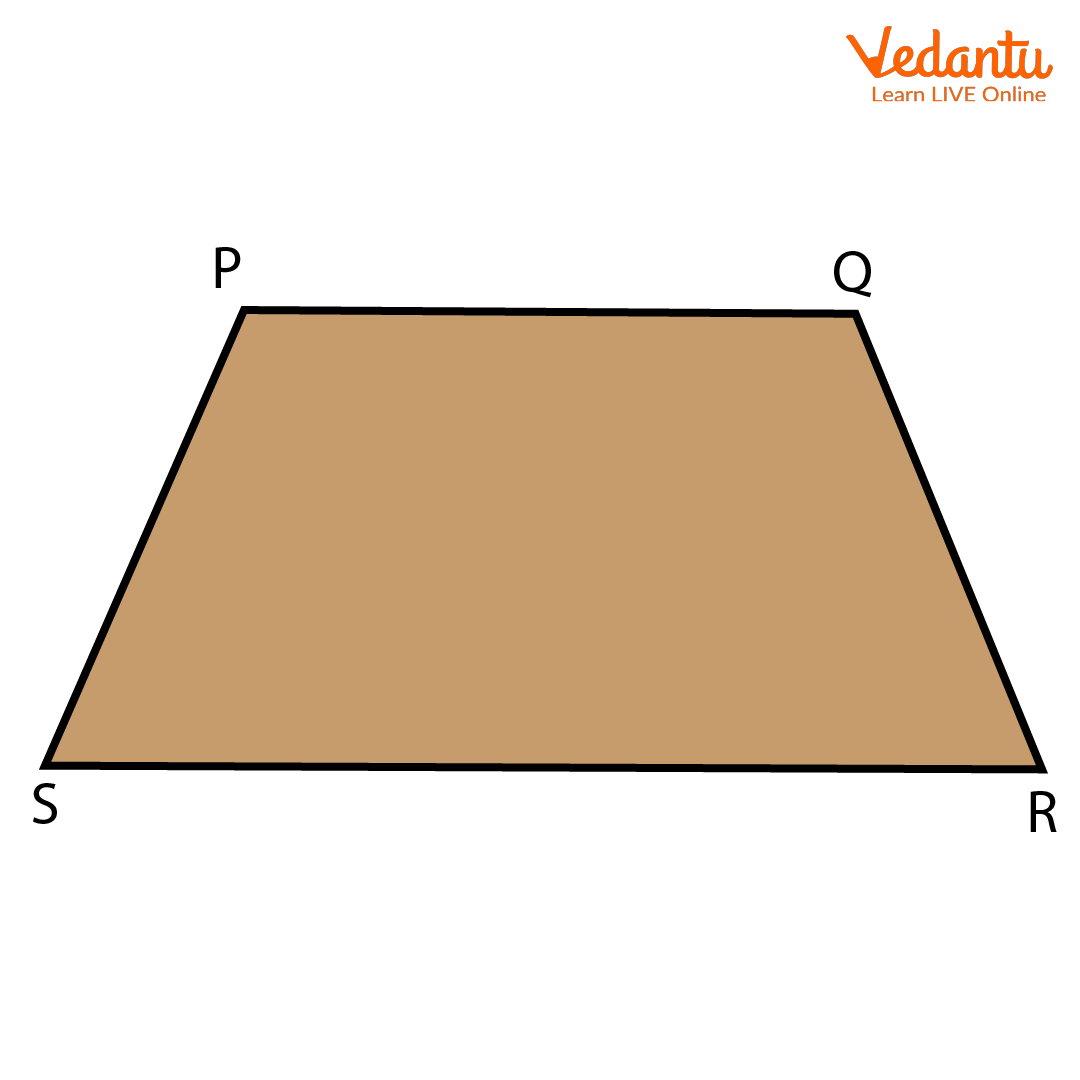Solution: In the given quadrilateral PQRS, (PQ, QR), (QR, RS), (RS, SP) and (SP, PQ) are the pairs of adjacent sides.

### Conclusion:

Thus, a quadrilateral is a closed figure with 4 vertices, 4 sides, 4 angles and 2 diagonals. A quadrilateral has 4 pairs of adjacent sides and 2 pairs of opposite sides. Talking about the angles, it has 2 pairs of opposite angles and 4 pairs of adjacent angles. Examples of quadrilaterals are Square, Rectangle, Rhombus, Parallelogram, Kite and Trapezium.

Last updated date: 30th Sep 2023
Total views: 70.2k
Views today: 1.70k

1. Can a square and rectangle be considered a quadrilateral?

Yes, a square and a rectangle can be considered quadrilateral as both are closed figures consisting of four vertices,  four sides, and four angles, and also, the sum of the four angles is $360^\circ$.

2. Can a triangle be considered a quadrilateral?

No, a triangle cannot be considered a quadrilateral as it is a figure with only three sides.

3. What is the sum of all the angles of a quadrilateral?

The sum of a quadrilateral's angles is $360^\circ$.

No, there will be no such quadrilateral having all of its angles as obtuse angles. As an obtuse angle is an angle greater than $90^\circ$ .Therefore, in this case, the sum of all the angles of the quadrilateral will also exceed $360^\circ$, which is not acceptable.